# SAT II Physics : Motion in One Dimension

## Example Questions

### Example Question #1 : Linear Motion

Near the surface of the earth, a projectile is fired from a canon at an angle of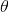degrees above the horizon and an initial velocity of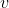meters per second. Which of the following expressions gives the time it takes the projectile to reach its maximum height?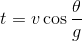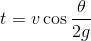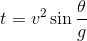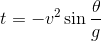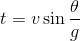Explanation:

At the maximum height of projectile motion,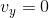and because this takes place near the surface of the earth we know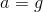which we can plug into the equation: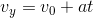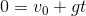We can then rearrange for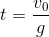Next substitute this value into the equation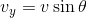to get the correct answer